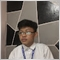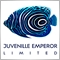# Grid trading, help with code16

HI, I am a senior high school student and I am new to trading and mt4 and ive been studying different financial market for the last 6 months. this grid system has a 60-70 percent winr ate and have a high gains in a day. this system doesn't use any indicator and news or fundamentals because indicator can be manipulated and news can give traders a false signal or etc.

this is a grid system. the strategy works by placing a order ex(Buy trade) and if the order hits take profit. it will open another buy order else if the order hits stop loss. it will open the opposite order ex(Sell Trade) and double the lot size.

problem:

code and broker

code:

the code of this EA is garbage because I am not efficient on the way i code hehe

the problem is the martingale. I don't know how to code martingale that will automatically double the lot size if the order hits the stop loss

suggestion:

i need a martingale code for this EA to work and I want to learn on how

```//+------------------------------------------------------------------+
//|                                                     GridTPLS.mq4 |
//|                         Copyright 2021, Karl Robeck Alferez    . |
//|                                             https://www.mql5.com |
//+------------------------------------------------------------------+
#property version   "1.00"
#property strict
#include "setlotsize.mq4"
input double lotsizeint=0.01;
extern int GridGap=100;
input double ATRMULTIPLIER=1.5;
input int magicnumber=5272003;

//+------------------------------------------------------------------+
//| Expert initialization function                                   |
//+------------------------------------------------------------------+
int OnInit()
{
if (OrdersTotal()==0)
{
int sellpos = OrderSend(_Symbol,OP_SELL,lotsizeint,Bid,3,Bid+GridGap*_Point,Bid-GridGap*_Point,NULL,magicnumber,0,Red);
//---
}
return(INIT_SUCCEEDED);
}
//+------------------------------------------------------------------+
//| Expert deinitialization function                                 |
//+------------------------------------------------------------------+
void OnDeinit(const int reason)
{
//---

}
//+------------------------------------------------------------------+
//| Expert tick function                                             |
//+------------------------------------------------------------------+
string signal="";
double Lotsize=setlotsize();
void OnTick()
{

signal=checkentrysignal();
// if we have sell signal
if(OrdersTotal()==0&&(signal=="sell"))
{
int sellpos = OrderSend(_Symbol,OP_SELL,lotsizeint,Bid,3,Bid+GridGap*_Point,Bid-GridGap*_Point,NULL,magicnumber,0,Red);
}
signal=checkentrysignal();
// if we have buy signal
{
}
signal=checkentrysignal();
// if we have buy martingale signal
{
}
// if we have sell martingale signal
signal=checkentrysignal();
if(OrdersTotal()==0&&(signal=="sellmg"))
{
int sellmgpos = OrderSend(_Symbol,OP_SELL,setlotsize(),Bid,3,Bid+GridGap*_Point,Bid-GridGap*_Point,NULL,magicnumber,0,Red);
}

}

// if we have position
string checkentrysignal()
{
int i=OrdersHistoryTotal()-1;
if(OrderSelect(i,SELECT_BY_POS,MODE_HISTORY)==true)
// if we have sell position
if(OrderType()==OP_SELL)
if(OrderMagicNumber()==magicnumber)
{
double OCP=OrderClosePrice();
if (OCP==OrderStopLoss())
{
}
// if takeprofit is reach continue sell grid
if (OCP==OrderTakeProfit())
{
signal="sell";
}
}
// if we have buy position
if(OrderMagicNumber()==magicnumber)
{
double OCP=OrderClosePrice();
// if takeprofit is reach continue buy grid
if (OCP==OrderTakeProfit())
{
}
// if stoploss is reach go opposite
if (OCP==OrderStopLoss())
{
signal="sellmg";
}
}
return signal;

}

```
```//+------------------------------------------------------------------+
//|                                                   setlotsize.mq4 |
//|                        Copyright 2021, MetaQuotes Software Corp. |
//|                                             https://www.mql5.com |
//+------------------------------------------------------------------+
#property version   "1.00"
#property strict

extern double first=0.01;
extern double second=0.02;
extern double third=0.04;
extern double forth=0.08;
extern double fifth=0.16;
extern double sixth=0.32;
extern double seventh=0.64;
extern double eight=1.28;
extern double nineth=2.56;
extern double tenth=5.12;
extern double eleventh=10.24;
extern double twelveth=20.48;
extern double thirteenth=40.96;
extern double fortheenth=81.92;
extern double fifthteenth=163.84;
extern double sixteenth=327.68;

double setlotsize()
{
int i=OrdersHistoryTotal()-1;
if(OrderSelect(i,SELECT_BY_POS,MODE_HISTORY)==true)

if(OrderMagicNumber()==magicnumber)
{
Lotsize=second;
}
if(OrderMagicNumber()==magicnumber)
{
Lotsize=third;
}
if(OrderMagicNumber()==magicnumber)
{
Lotsize=forth;
}
if(OrderMagicNumber()==magicnumber)
{
Lotsize=fifth;
}
if(OrderMagicNumber()==magicnumber)
{
Lotsize=sixth;
}
if(OrderMagicNumber()==magicnumber)
{
Lotsize=seventh;
}
if(OrderMagicNumber()==magicnumber)
{
Lotsize=eight;
}
if(OrderMagicNumber()==magicnumber)
{
Lotsize=nineth;
}
if(OrderMagicNumber()==magicnumber)
{
Lotsize=tenth;
}
if(OrderMagicNumber()==magicnumber)
{
Lotsize=eleventh;
}
if(OrderMagicNumber()==magicnumber)
{
Lotsize=twelveth;
}
if(OrderMagicNumber()==magicnumber)
{
Lotsize=thirteenth;
}
if(OrderMagicNumber()==magicnumber)
{
Lotsize=fortheenth;
}
if(OrderMagicNumber()==magicnumber)
{
Lotsize=fifthteenth;
}
if(OrderMagicNumber()==magicnumber)
{
Lotsize=sixteenth;
}
if (signal=="sellmg" && OrderLots()==first)
if(OrderMagicNumber()==magicnumber)
{
Lotsize=second;
}
if (signal=="sellmg" && OrderLots()==second)
if(OrderMagicNumber()==magicnumber)

{
Lotsize=third;
}
if (signal=="sellmg" && OrderLots()==third)
if(OrderMagicNumber()==magicnumber)
{
Lotsize=forth;
}
if (signal=="sellmg" && OrderLots()==forth)
if(OrderMagicNumber()==magicnumber)
{
Lotsize=fifth;
}
if (signal=="sellmg" && OrderLots()==fifth)
if(OrderMagicNumber()==magicnumber)
{
Lotsize=sixth;
}
if (signal=="sellmg"&& OrderLots()==sixth)
if(OrderMagicNumber()==magicnumber)
{
Lotsize=seventh;
}
if (signal=="sellmg"&& OrderLots()==seventh)
if(OrderMagicNumber()==magicnumber)
{
Lotsize=eight;
}
if (signal=="sellmg"&& OrderLots()==eight)
if(OrderMagicNumber()==magicnumber)
{
Lotsize=nineth;
}
if (signal=="sellmg"&& OrderLots()==nineth)
if(OrderMagicNumber()==magicnumber)
{
Lotsize=tenth;
}
if (signal=="sellmg"&& OrderLots()==tenth)
if(OrderMagicNumber()==magicnumber)
{
Lotsize=eleventh;
}
if (signal=="sellmg"&& OrderLots()==eleventh)
if(OrderMagicNumber()==magicnumber)
{
Lotsize=twelveth;
}
if (signal=="sellmg"&& OrderLots()==twelveth)
if(OrderMagicNumber()==magicnumber)
{
Lotsize=thirteenth;
}
if (signal=="sellmg"&& OrderLots()==thirteenth)
if(OrderMagicNumber()==magicnumber)
{
Lotsize=fortheenth;
}
if (signal=="sellmg"&& OrderLots()==fortheenth)
if(OrderMagicNumber()==magicnumber)
{
Lotsize=fifthteenth;
}
if (signal=="sellmg"&& OrderLots()==fifthteenth)
if(OrderMagicNumber()==magicnumber)
{
Lotsize=sixteenth;
}
if (signal=="sell")
if(OrderMagicNumber()==magicnumber)
Lotsize=first;
if(OrderMagicNumber()==magicnumber)
Lotsize=first;

return Lotsize;
}```
Files:Moderator
20458

You can get help with your code, but discussion about brokers is not allowed in this forum.16

Eleni Anna Branou #:
You can get help with your code, but discussion about brokers is not allowed in this forum.

ok i will remove it tnx26425

Karl Robeck: i need a martingale code for this EA to work and I want to learn on how

Hedging, grid trading, same as Martingale.
Martingale, Hedging and Grid : MHG - General - MQL5 programming forum (2016)

Martingale, guaranteed to blow your account eventually. If it's not profitable without, it is definitely not profitable with.
Martingale vs. Non Martingale (Simplified RoR vs Profit and the Illusions) - MQL5 programming forum (2015)16

William Roeder #:

Hedging, grid trading, same as Martingale.
Martingale, Hedging and Grid : MHG - General - MQL5 programming forum (2016)

Martingale, guaranteed to blow your account eventually. If it's not profitable without, it is definitely not profitable with.
Martingale vs. Non Martingale (Simplified RoR vs Profit and the Illusions) - MQL5 programming forum (2015)

tnx mate i will read it